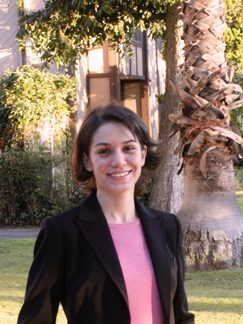Maryam Fazel# Maryam Fazel

### Electrical Engineering Department, University of Washington

University of Washington
Department of Electrical and Computer Engineering
Paul Allen Center - Room AE100R
Campus Box 352500
Seattle, WA 98195-2500
Fax: (206) 543-3842
E-mail:
mfazel (at) ee(dot)washington(dot)edu
URL:
http://www.ee.washington.edu/~mfazel

I am an Associate Professor in ECE, and hold adjunct appointments in the departments of Computer Science and Engineering, Mathematics, and Statistics at UW. My research interests are in convex optimization, algorithms, machine learning, and control. Prior to joining UW EE, I was a Research Scientist at Caltech. I received my PhD in Electrical Engineering from Stanford University where I was part of the Information Systems Lab, advised by Prof. Stephen Boyd. I co-direct our new NSF TRIPODS Institute, ADSI. I serve on the Editorial board of the MOS-SIAM Book Series on Optimization, and am an Associate Editor of the SIAM Journal of Optimization (SIOPT), and an associate editor of the new SIAM Journal on Mathematics of Data Science (SIMODS). I am also the chair of the annual ECE Lytle Lecture Series committee, and a coorganizer of the interdepartmental CORE Seminar Series.

• My entry in the Mathematics Genealogy Project
• My Erdos number is 3!

### Teaching

**** EE/AA/ME 578: Optimization in System Sciences ****
Winter quarter 2008, Univ of Washington
Course webpage COMING SOON!

### Education

• Ph.D. Electrical Engineering, Stanford University, CA, March 2002
• M.S. Electrical Engineering, Stanford University, CA, June 1997
• B.Sc. Electrical Engineering, Sharif University of Technology, Tehran, Iran, 1995

### Research Interests

• Optimization, in particular convex optimization; convex relaxations and approximations; algebraic methods such as Sum of Squares
• Systems and Control; Linear Matrix Inequalities
• Finding ``simple" models (or Parsimonious Modeling) and Matrix Rank Minimization; convex relaxations for rank minimization
• Compressed sensing and sparse signal recovery
• Network resource allocation; distributed optimization
• Applications in: Networking, Systems Biology, Physiological Modeling

### Ph.D. Thesis

• Matrix Rank Minimization with Applications. Elec. Eng. Dept, Stanford University, March 2002.
Thesis: (ps file), (pdf file), (gzipped ps file)

My PhD work concerns the problem of minimizing the rank of a matrix over a convex set. In many engineering applications, notions such as order, complexity, or dimension of a model or design can be expressed as the rank of an appropriate matrix. If the set of feasible models or designs is convex, then choosing the simplest model can be cast as a Rank Minimization Problem (RMP). For example, a low-rank matrix could correspond to a low-order controller for a plant, a low-degree statistical model fit for a random process, a shape that can be embedded in a low-dimensional space, or a design with a small number of components. It is thus not surprising that rank minimization has diverse applications: following Occam's razor, we often seek simple explanations and designs.

The RMP is known to be NP-hard. If the variable in an RMP is a positive semidefinite matrix, a simple and effective relaxation commonly used is trace minimization. We prove that any general RMP involving non-positive semidefinite or even non-square matrices, can be embedded in a larger, positive semidefinite RMP. This semidefinite embedding lemma is then used to extend the trace relaxation, as well as other methods using semidefiniteness, to general matrices. The generalized trace relaxation minimizes the dual of the matrix 2-norm, referred to as the nuclear norm. Furthermore, we show that this relaxation is equivalent to minimizing the convex envelope of the rank function over the unit ball, thus providing theoretical support for its use. We also propose another heuristic based on (locally) minimizing the logarithm of the determinant (log-det) of the matrix, to refine the result of the trace relaxation and reduce the rank further. This heuristic is in fact equivalent to iteratively minimizing a weighted trace objective, and thus also admits an interpretation in terms of convex envelopes. Finally, applying these methods to application examples shows they work well in practice.

Related papers:

*** Page under construction! ***